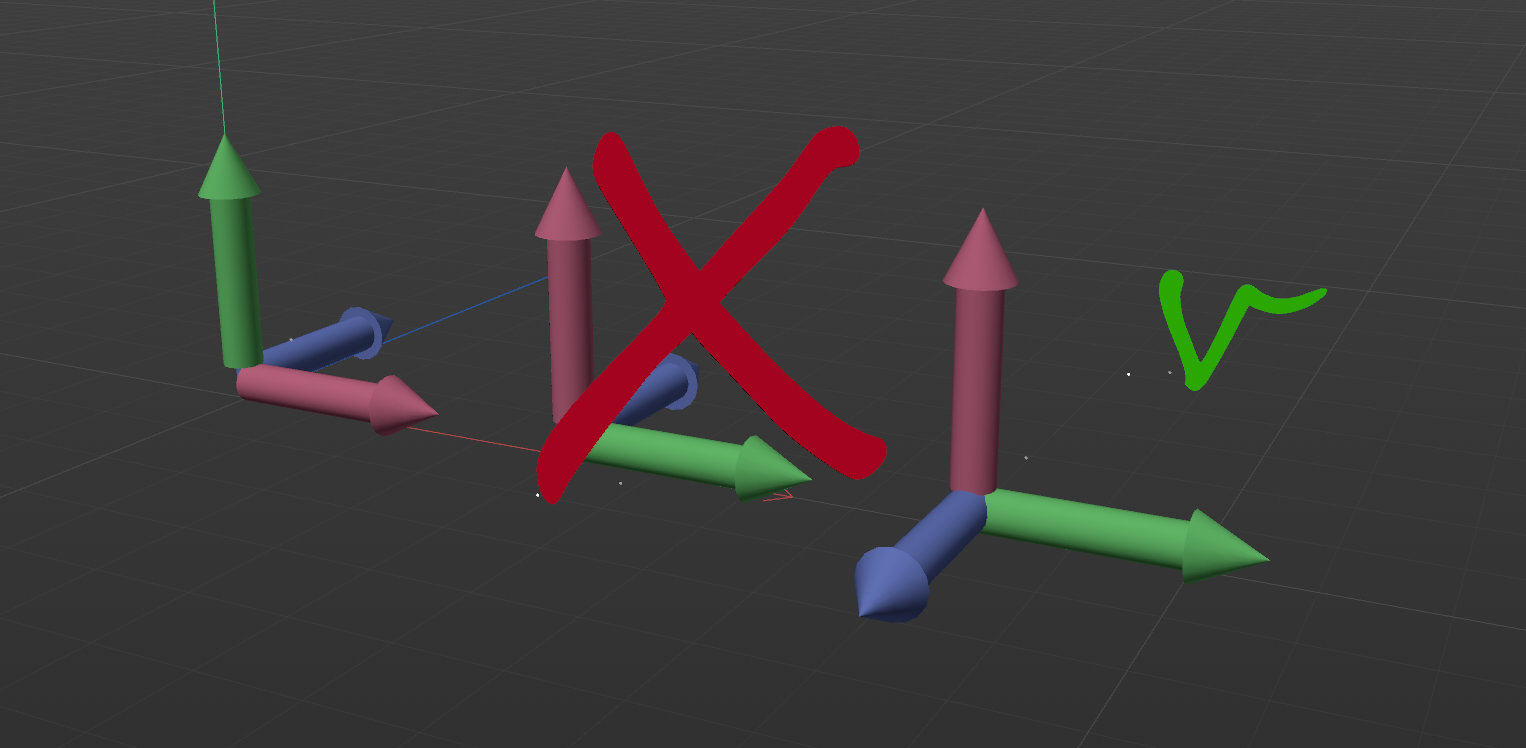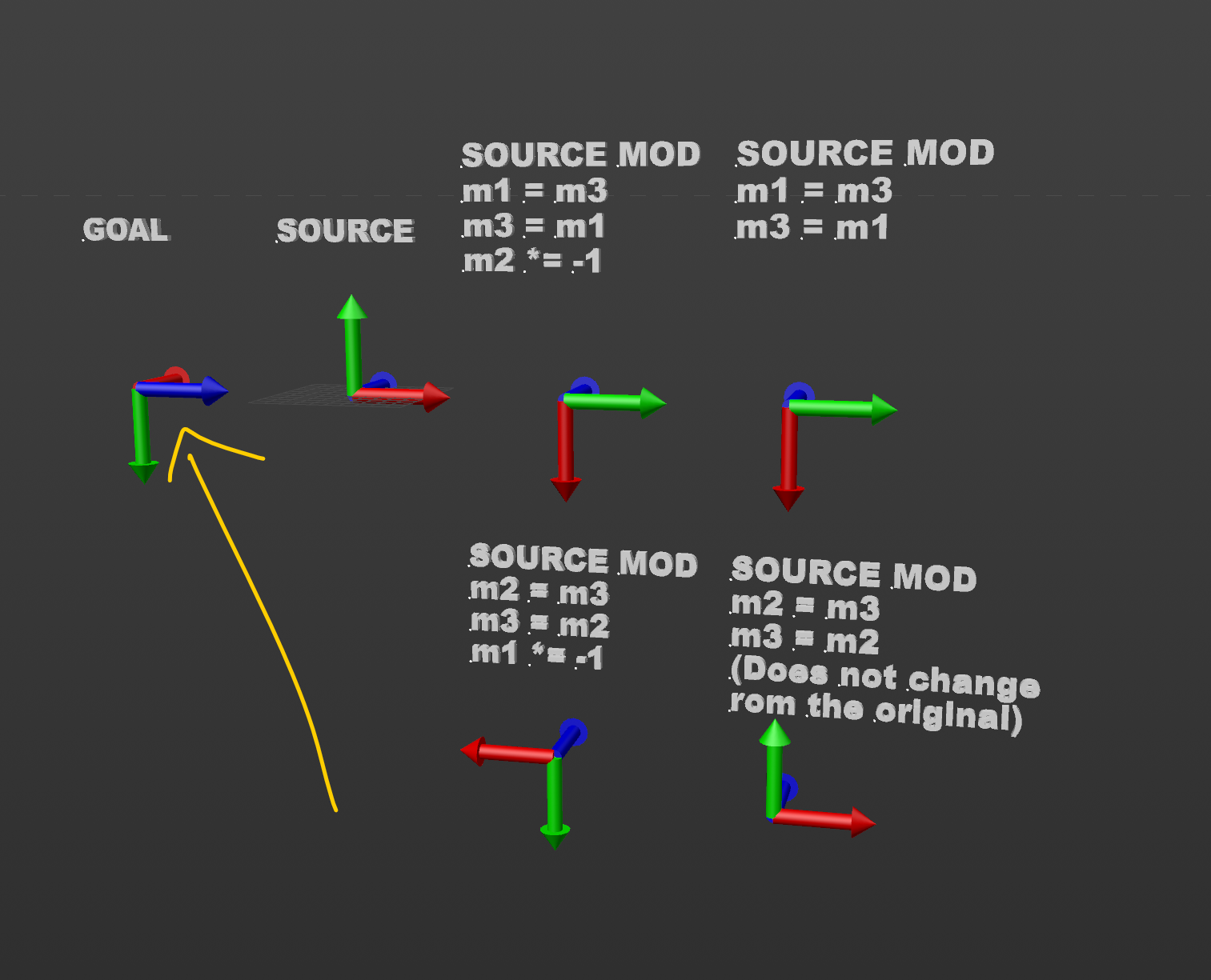# SOLVEDSwap Out Joint Axis Rotation?

Is there a handy way to swap out joint axis rotation? Like some API command *wink *wink

For example, if the skeleton structure aim axis is at X axis, I want it to be Z axis. And correspondingly swap out the up-axis too.

Currently, what I looking forward is:

1. Store Skeleton Hierarchy Info
2. Unparent joints resulting to a flat hierarchy
3. Do a manual axis rotation swapping (pending an answer on this thread)
4. Reparent joints based on #1 Skeleton Hierarchy Info.

But I can already see a lot of point of failure especially on complex hierarchy which rigs mostly have.

P.S. I can't use the joint axis align tool because it recomputes the rotation. It might be aiming to the proper axis but the up axis is all out of whack. haha. Doing a manual set-up of each joint's up axis defeats the pointHi,

to swap axis using matrices, you just need to swap the vector values corresponding to the axis you want to swap. Of course, you have to be careful that the swapping will not create something else than a left-hand coordinate system.

For example, if you want to swap X and Y asix, Z must look at the opposite direction. The middle example is a right-hand coordinate system.in that case, with the matrix m, m.v1 = m.v2, m.v2 = m.v1 and m.v3 *= -1.

About the hierarchy, you must update the first child and calculate its new position/rotation. That is done by using matrix calculation (that you might know)

Cheers,
Manuel

@manuel

Thanks for the illustrated response. It does work with X,Y. But swapping with Z, it does not seem to work, which is what I'm after.

You can check in the image below, none of them are copying the Z axis even if swapping to the X or Y axisi forgot to say that you must use a temporary variable to store the value, otherwise you cannot swap them. I am sure it is not the issue but just to be sure.

I am using that code.

``````    mg = op.GetMg()
temp = mg.v3 # keeping track of where the z axis is pointing to
mg.v3  = mg.v1 # make the z axis points to the same direction as x
mg.v1 = temp # make x pointing to where z was pointing
mg.v2 *= -1 # make y pointing the opposite direction of where it was pointing to
op.SetMg(mg)
``````

Cheers,
Manuel

@manuel

Just used your code and it works as expected.

But the thing is we actually have more or less the same code.

``````    m = op.GetMg()
m_copy = m
m.v1 = m_copy.v3
m.v3 = m_copy.v1
m.v2 *= -1
op.SetMg(m)
``````

The only difference is that I'm storing a copy of the whole matrix and while you are storing a copy of a vector.

It should work the same code as yours right?

If you want a copy of a matrix, you must use the copy constructor otherwise, the vector will be references and not new variables.

``````    m = op.GetMg()
m_copy = c4d.Matrix(m)
m.v1 = m_copy.v3
m.v3 = m_copy.v1
m.v2 *= -1

``````

Cheers,
Manuel

@manuel

Interesting. Thanks for the heads up.
It works now as expected.